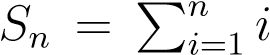1. Math
2. Advanced Math
3. i need help with these please problem 4 calculate 1...

# Question: i need help with these please problem 4 calculate 1...

###### Question details

I need help with these please.

Problem 4.

Calculate

1 + 2 + 3 + ··· + 98 + 99 + 100

by deriving a general formula for. To that end start with the conjectural form for Sn = an2 + bn + c with some unknown a,b,c and then fix these coefficients by considering Sn for different values of n.

Problem 5.

Check the expression forby showing that (1 + x) × (1 + x)a = (1 + x)a+1. If the case of general a poses challenge, check this expression for a = 3.

###### Solution by an expert tutor